# Decision Trees and Random Forests

dougieduk·2022년 4월 2일
0

# Elements of a decision tree

• Nodes
- Split for the value of a certain attribute

• Edges
- Outcome of a split to next node

• Root
- The node that performs the first split

• Leaves
- Terminal nodes that predict the outcome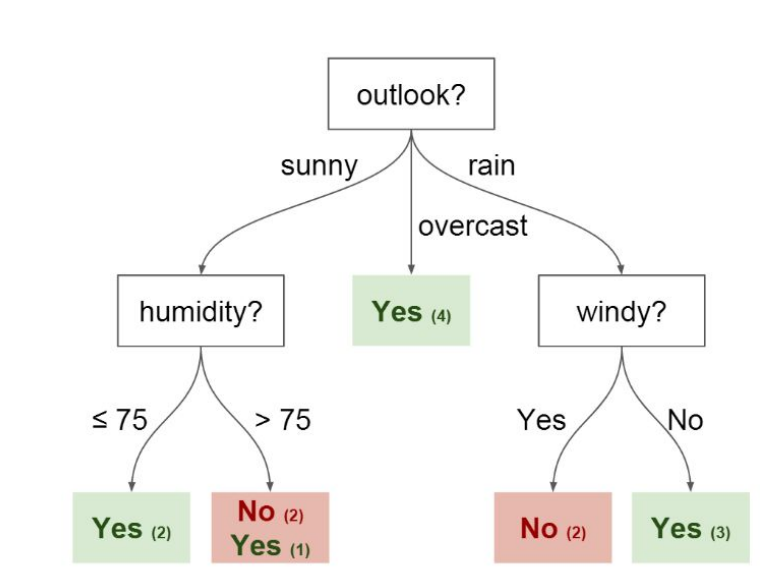# Intuitions behind the split

• We try to choose the variables which splits the tree most cleanly

# Concept of Impurity

• How do you define clean?
- Entropy and Information Gain are the Mathematical Methods of choosing the best split. Refer to reading assignment
• Information gain is the (Beginning entropy )- (sum of the entropy of the terminal nodes)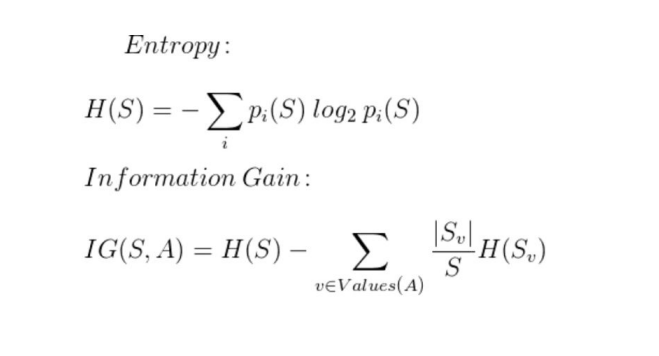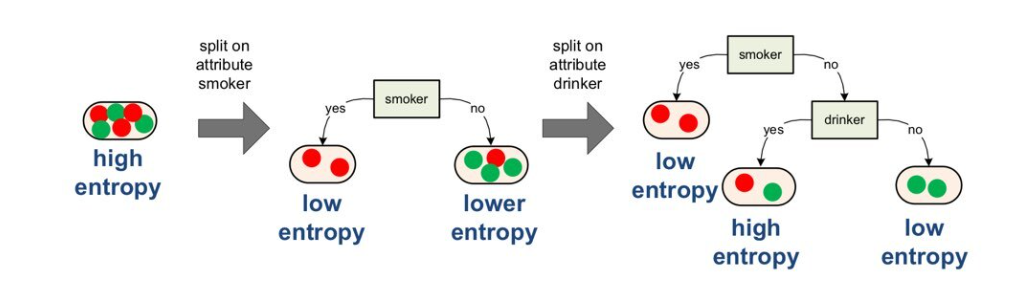• Example of Entropy
- There's a node with 3 reds, 3 greens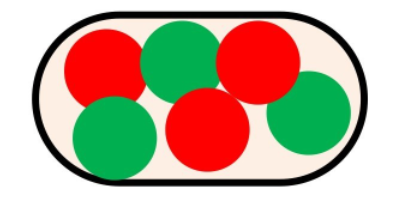- Entropy is calculated as below: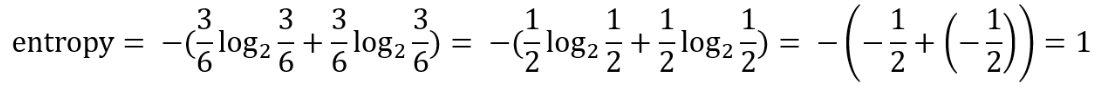• GINI Index could also be used:
- How to calculate GINI Index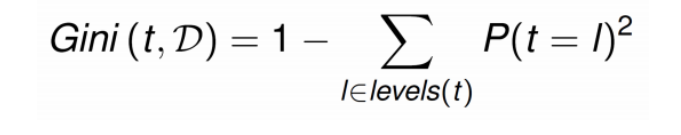- Calculating a GINI Index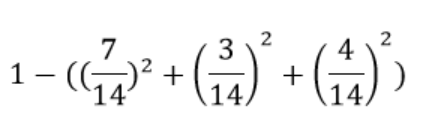# Information Gain

• Calculating Information Gain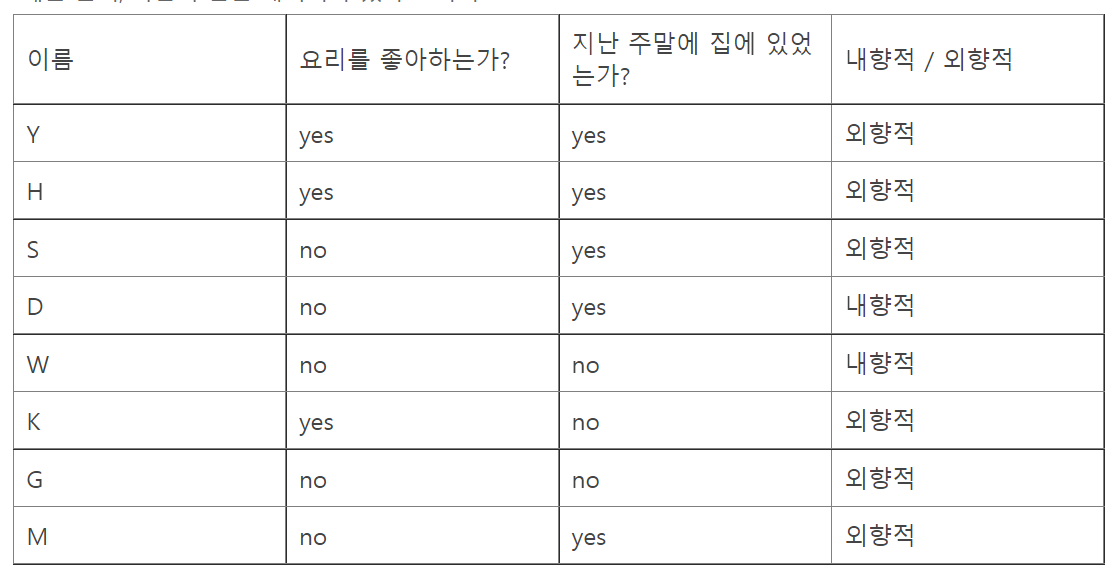- Beginning Entropy :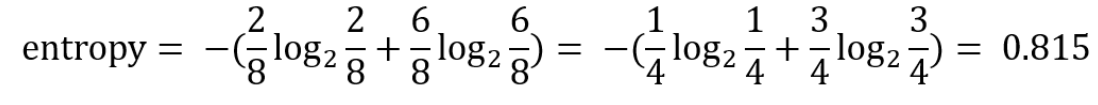- Entropy of the leaf nodes :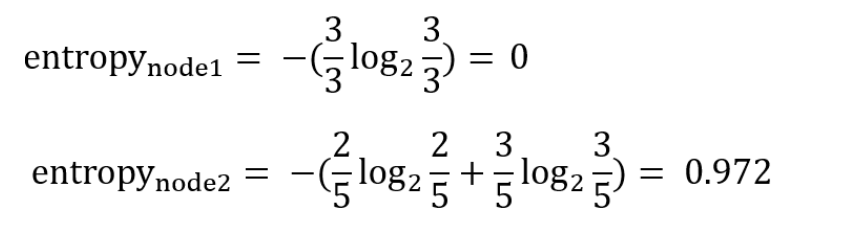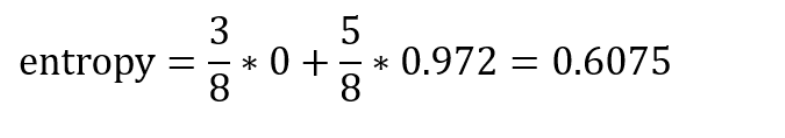- Information Gain :
0.815-0.6075 = 0.2075

• We repeat this process until the information gain is less than a certain threshold (e.g. 0.1)

# Information Gain Ratio (GR)

• The more nodes you create , higher is the Information Gain. But is this a good model?
- That's where GR comes in

• How you calculate GR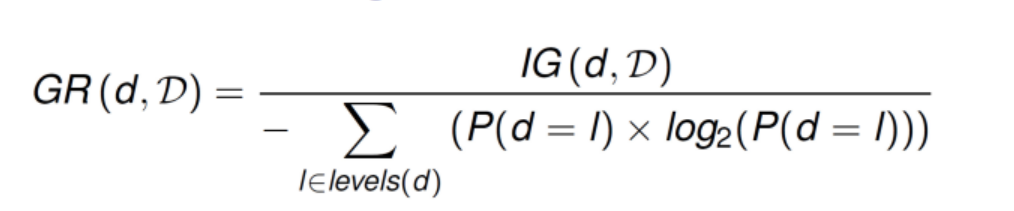• Example
- an example model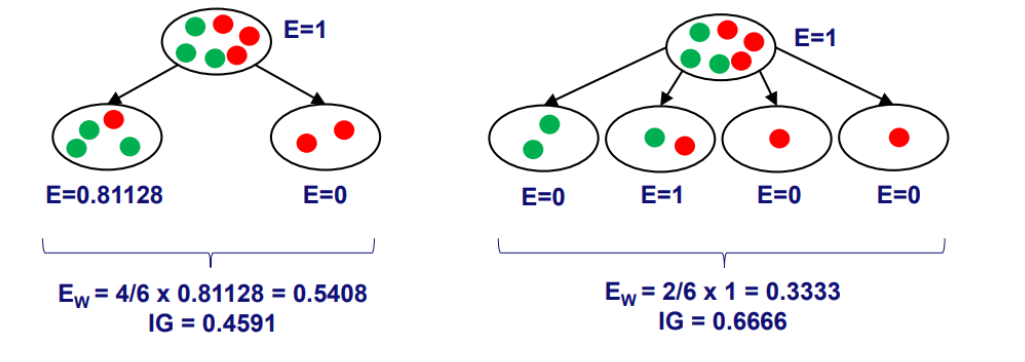- Denominator of each model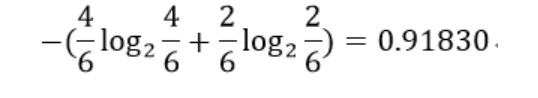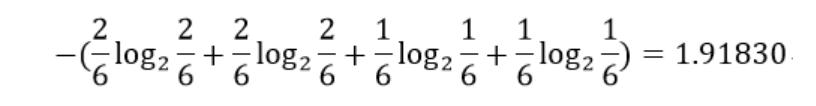- Calculating GR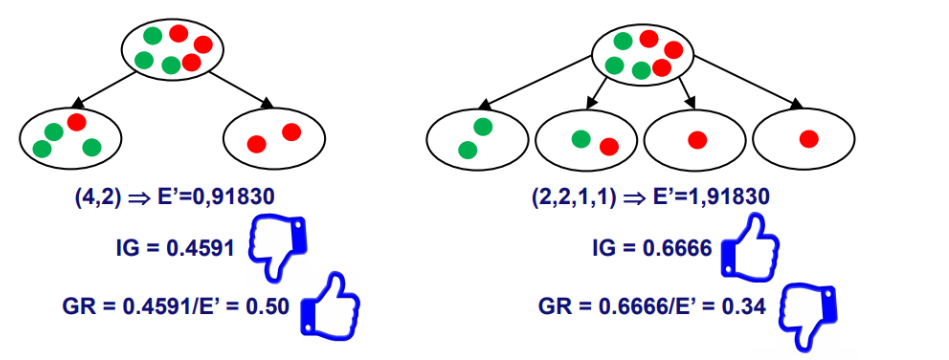# Random Forest

• Decision Trees tend to overfit. Therefore you create multiple decision trees and let the trees do the voting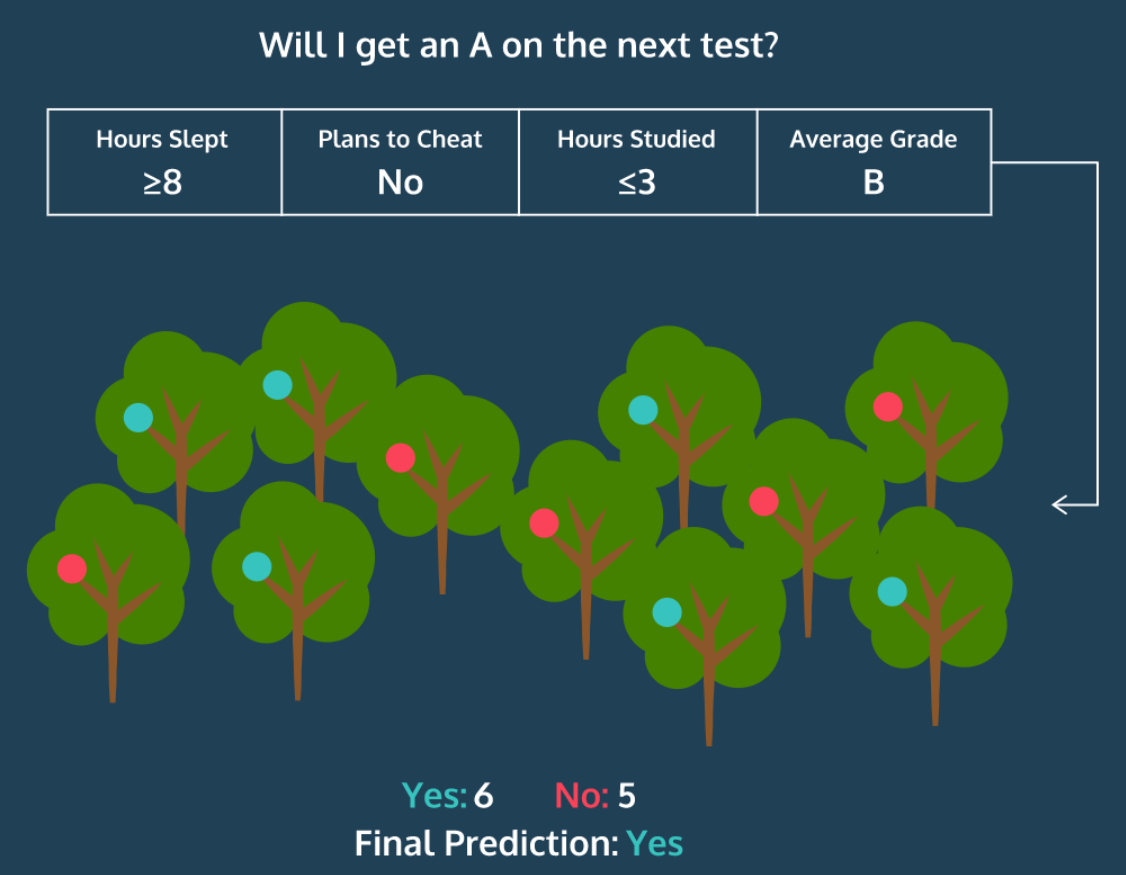• This is one of Ensemble Machine Learning Methods

# Bagging

• Selecting random features/ rows from data with replacement

• Bagging Features
- If you choose the features to split on, like the traditional decision tree model, the models are likely to start spliting with the same feature (the strongest feature)

• In this case, the trees are likely to be highly correlated
• Therefore, you randomly choose the features
• number of features to choose is the sqrt of total no. of features
• Bagging Rows
- You also do the same for rows

The Contents of this post belongs to Jose Portilla's Python for Data Science and Machine Learning Bootcamp and 유나의 공부